Home Articles Malaysian Tiungsat-1 Imagery for Water Quality Mapping

# Malaysian Tiungsat-1 Imagery for Water Quality Mapping

M. Z. MatJafri, K. Abdullah, H. S. Lim
School of Physics
Universiti Sains Malaysia
11800, Pulau Pinang.
Tel: 604-6577888 Fax: 604-6579150
E-mail: [email protected] and [email protected]

1. Introduction
Suspended matter deposited in reservoirs reduces their storage capacity and suspended material reduces the light penetration in water, thus minimizing the fish production (Choubey, 1998). The major factors affecting surface water quality are suspended sediments, chlorophyll, nutrients and pesticides. The standard traditional mapping and monitoring techniques have already become too expensive compared to the information achieved for environmental use. A solution could be to optimize our efforts and more frequently base our surveillance on remote sensing techniques to improve the information content and limit the cost (Ostlund 2001). Remote sensing offers potentially a significant source of information and methods are being developed for operational large-scale monitoring of water quality (Koponen et al. 2002).

In this study, satellite scene from Tiungsat-1 was used for water quality mapping around Penang Island. This preliminary study indicates the possibility of using Tiungsat-1 satellite image for monitoring coastal water quality.

Multi-spectral algorithms were used to determine the TSS concentration on the surface of seawater. Various algorithms were also tested and the values of their correlation coefficient (R) and root-mean-square deviation (RMS) were compared. The proposed algorithm was used to generate the water quality map. Finally, the digital image was geometrically corrected to produce a water quality map.

2. Study Area
The study area is located between latitudes 5° 12′ N to 5° 30′ N and longitudes 100° 09′ E to 100° 26′ E as shown in Figure 1. The Tiungsat-1 satellite image captured on 17 March 2001 was used in the present investigation. The water sample data collected within the region of interest were available for calibration of the quality algorithm.

3. Optical Model of Water
A physical model relating radiance from the water column and the concentrations of the water quality constituents provide the most effective way of analyzing remotely sensed data for water quality studies. Reflectance is particularly dependent on inherent optical properties: the absorption coefficient and the backscattering coefficient. The irradiance reflectance just below the water surface, R(l), is given by Kirk (1984) as
R(l) = 0.33b(l)/a(l)           (1)

where
l = the spectral wavelength
b = the backscattering coefficient
a = the absorption coefficientFigure 1. The location of the study area
The inherent optical properties are determined by the contents of the water. The contributions of the individual components to the overall properties are strictly additive (Gallegos and Correl, 1990).

For a case involving two water quality components, i.e. chlorophyll, C, and suspended sediment, P, the simultaneous equations for the two channels given by Gallie and Murtha (1992) can be expressed as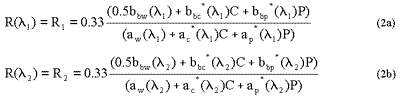where
bbw(i) = backscattering coefficient
bbc*= chlorophyll coefficient
bbp = sediment coefficient
aw(i)= absorption coefficient
ac*= chlorophyll specific absorption coefficient
ap*= sediment specific absorption coefficient
C= chlorophyll
P= suspended sediment

4. Regression Algorithm
TSS concentration can be obtained by solving the two simultaneous equations to obtain the series of terms R1 and R2 that is given as
P = ao+a1R1+a2R2+a3R1R2+ a4R12+ a5R22+ a6R12R2+ a7R1 2R22+a8R12R22+…         (3)
Where aj, j = 0, 1, 2, … are the coefficient for equation (3) that can be solved empirically using multiple regression analysis. This equation can also be extended to the three-band method given as
P = eo+e1R1+e2R2+e3R3+ e4R1R2+ e5R1R3+ e6R2R3+ e7R12+e8R22+e9R32         (4)
where the coefficient ej, j = 0, 1, 2, … can also be solved empirically.

5. Data Analysis and Result
A Tiungsat-1 satellite scene of the study area contained 1024 x 1024 pixels. The image contained band-to-band registration error and also systematic banding. In this study, the three-band images were shifted accordingly so that the three bands overlap each other. An image size of 420 x 429 pixels was then extracted covering the study area. Table 1 shows the parameters of the Tiungsat-1 scene and Table 2 shows the Tiungsat-1 sensor characteristics for bands 1 to 3. The spatial resolution of the image pixel is 78 m (Astronautic Technology, 2002).
Table 1. Scene parameters of Tiungsat-1 used in this study

 Location Penang Date 17-3-2001 Time 15:31:28 Sun Elevation 58.3 Sun Azimuth 258.8 Latitude (°) 5.363 Longitude (°) 100.309

Table 2. Tiungsat-1 sensor characteristics for band 1 to 3

 Band Wavelength (nm) Nominal spectral band 1 510-590 Green 2 610-690 Red 3 810-890 Near-infrared

The best Tiungsat-1 image available for the study area did not have the coincident data of total suspended solid. To overcome this problem we correlated the Tiungsat-1 image with the Landsat TM images that have the corresponding sea-truth data. All the three bands of the images ware correlated and examined. The analysis revealed that the Landsat TM image of 20 January 2000 produced the highest correlation with the Tiungsat-1 image. Then the data collected on 20 January 2000 were used in the present study. Figure 2-4 show the DNs correlation between red, green, and infrared bands of Tiungsat-1 image with the corresponding TM bands.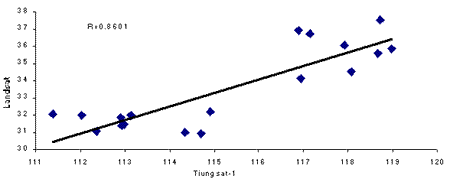Figure 2. Graph of DNs relationship between Landsat TM image and Tiungsat-1 image for red band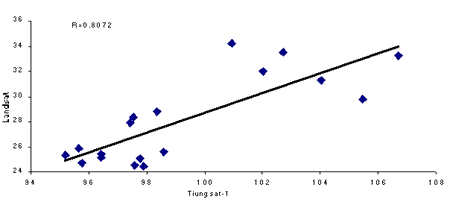Figure 3. Graph of DNs relationship between Landsat TM image and Tiungsat-1 image for green band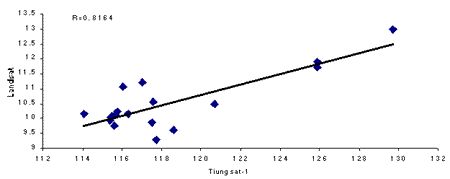Figure 4. Graph of DNs relationship between Landsat TM image and Tiungsat-1 image for infrared band

The digital numbers (DN) for each band corresponding to the sea-truth locations were determined. The station locations were computed using the second order polynomial transformation equations determined from ground control points (GCP). Digital numbers were determined for each band using different window sizes, such as, 1 by 1, 3 by 3, 5 by 5, 7 by 7, 9 by 9 and 11 by 11. The DNs values extracted using the window size of 7 by 7 were used due to the higher correlation coefficient (R) with the sea-truth data.

The data shows positive correlations between DN and TSS. Such characteristics were also observed by other investigators using remote sensing data in the visible channel for suspended sediment studies (Collins and Pattiaratshi, 1984, Schiebe et al., 1992, Choubey, 1992).

In this study, raw DN values were used as independent variables in our calibration regression analyses. Other forms of water quality algorithms were tested with the data set and their accuracies were compared with that of the proposed algorithm. For each regression model the correlation coefficient, R, and the root-mean-square deviation, RMS, were noted. Many investigators used these parameters in their studies (Keiner and Yan, 1998, Pulliainen et al., 2001). Table 3 shows the comparative performance of the algorithm. The proposed algorithm produced higher correlation coefficient between the predicted and the measured TSS values and lower RMS values compared to other algorithms. With the present data set, the R and RMS values were 0.9256 and 3.6237 mg/l respectively as indicated in Figure 5.
Table 3. Regression results using different forms of algorithms for TSS

 Algorithm R RMS TSS=a0+a1B1+a2B12 0.6334 7.4167 TSS=a0+a1B2+a2B22 0.6305 7.4348 TSS=a0+a1B3+a2B32 0.4098 8.7489 TSS=a0+a1lnB1+a2lnB12 0.6406 7.7235 TSS=a0+a1lnB2+a2lnB22 0.6320 7.5200 TSS=a0+a1lnB3+a2lnB32 0.4083 8.7403 TSS=a0+a1(B1/B3)+a2(B1/B3)2 0.6168 7.5340 TSS=a0+a1(B1/B2)+a2(B1/B2)2 0.3878 8.8225 TSS=a0+a1(B2/B3)+a2(B2/B3)2 0.3302 9.0349 TSS=a0+a1ln(B1/B3)+a2ln(B1/B3)2 0.6134 7.5590 TSS=a0+a1ln(B1/B2)+a2ln(B1/B2)2 0.3962 8.7883 TSS=a0+a1ln(B2/B3)+a2ln(B2/B3)2 0.3528 8.9558 TSS=a0+a1(B1-B3)/B2+ a2((B1-B3)/B2)2 0.6101 7.5842 TSS=a0+a1(B2-B3)/B1+ a2((B2-B3)/B1)2 0.4196 8.6880 TSS=a0+a1(B1-B2)/B3+ a2((B1-B2)/B3)2 0.3818 8.8464 TSS=a0+a1(B2-B1)/(B1+B2)+ a2(B2-B1)/(B1+B2)2 (Waldron M. C. et al.) 0.3969 8.7856 TSS=a0+a1(B2-B3)/(B2+B3)+ a2(B2-B3)/(B2+B3)2 (Waldron M. C. et al.) 0.3511 8.9620 TSS=a0+a1(B1-B3)/(B1+B3)+ a2(B1-B3)/(B1+B3)2 0.6136 7.5582 TSS=a0+a1(B1+B2)/2+ a2((B1+B2)/2)2 (Waldron M. C. et al.) 0.3558 8.9562 TSS=a0+a1(B1+B3)/2+ a2((B1+B3)/2)2(Waldron M. C. et al.) 0.4521 8.5345 TSS=a0+a1(B2+B3)/2+ a2((B2+B3)/2)2(Waldron M. C. et al.) 0.7096 6.7906 TSS=a0+a1B1+a2B2+a3B3+a4B1B2+a5B1B3+a6B2B3+a7B12+a8B22+a9B32 (Proposed) 0.9256 3.6237

Note: B1, B2 and B3 are the digital numbers for red, green and infrared bands respectively
A map of the water quality parameter was then generated using the calibrated proposed algorithm. Then the generated water quality map was geometrically corrected using the cubic convolution method to produce a smoother map. The generated map was filtered using 5 by 5 pixels averaged to remove random noise and then colour-coded for visual interpretation as shown in Figure 6.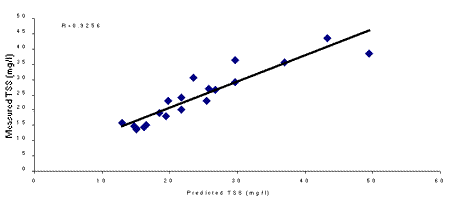Figure 5. Relationship between measured and predicted TSS
The local distribution pattern is shown on the generated TSS map. Higher concentration areas are distributed near the river mouths and the shallow southern region of the channel. The plumes in the river mouths were created by river discharges. In the shallow water, the turbidity might be caused by bottom resuspension due to waves and wind actions. The concentration seems to be relatively lower in the deeper part of the sea. The pattern produced in Figure 6 is consistent with the sea truth data as well as earlier reports (Din, 1995). However, we are unable to establish the validity of the apparent higher concentration on the west coast of Penang Island because of the absence of sea-truth data. Further sea truthing for the area will be done to confirm the values.

 Malaysian Tiungsat-1 Imagery for Water Quality Mapping 6. Conclusion Tiungsat-1 can provide a useful tool for water quality mapping around Penang Island. To improve the accuracy of the parameter, the sea-truth data are very important for determining the water quality. The sea-truth data on the date the satellite overpasses the study area must be collected and atmospheric correction has to be done for further analysis. The feasibility of applying the present technique for operational use has to be further validated. Therefore more data will be required for this verification analysis.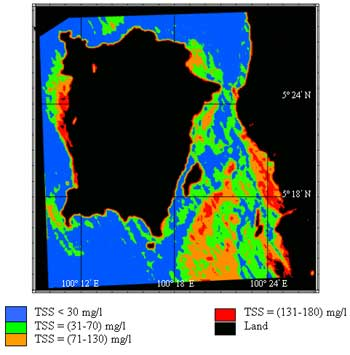Figure 6. TSS map around Penang Island Acknowledgments The authors would like to thank Astronautic Technology and Universiti Teknologi Malaysia for supplying the Tiungsat-1 image. References ASTRANAUTIC TECHNOLOGY, 2002, Projects Tiungsat-1, date visited; 25 June 2002. CHOUBEY V. K., 1998, Laboratory experiment, field and remotely sensed data analysis for the assessment of suspended solids concentration and secchi depth of the reservoir surface water. International Journal of Remote Sensing, 19, 3349-3360. COLLINS M. and PATTIARATCHI C., 1984, Identification of suspended sediment in coastal waters using airborne thematic mapper, International Journal of Remote Sensing, 5, 653-657. DIN, Z.B., 1995, Environmental impact assessment study of the proposed 275kV overhead cable crossing: Juru to Bayan Lepas, Final consultancy report submitted to the Tenaga National Berhad. GALLEGOS, C. L., and CORREL D. L., 1990, Modeling spectral diffuse attenuation, absorption and scattering coefficients in a turbid estuary. Limnology and Oceanography, 35, 1486-1502. GALLIE, E. A., and MURTHA, P. A., 1992, Specific absorption and backscattering spectra for suspended minerals and chlorophyll-a in Chilko Lake, British Columbia. Remote Sensing of Environment, 39, 103-118. KEINER, L. E., and XIAO, HAI YAN, 1998, A neural network model for estimating sea surface chlorophyll and sediments from thematic mapper imagery. Remote Sensing of Environment, 66, 153-165. KIRK, J. T. O., 1984, Dependence of relationship between inherent and apparent optical properties of water on solar altitude. Limnology and Oceanography, 29, 350-356. KOPONEN, S., PULLIAINEN, J., KALLIO, K., and HALLIKAINEN, M., 2002, Lake water quality classification with airborne hyperspectral spectrometer and simulated MERIS data. Remote Sensing of Environment, 79, 51-59. OSTLUND, C., FLINK, P., STROMBECK, N., PIERSON, D. and LINDELL, T., 2001, Mapping of the water quality of lake Erken, Sweden, from imaging spectrometry and Landsat Thematic mapper. The Science of The Total Environment, 268, 139-154. PULLIAINEN, J., KALLIO, K., ELOHEIMO, K., KOPONEN, S., SERVOMAA, H., HANNONEN, T., TAURIAINEN, S. and HALLIKAINEN, M., 2001, A semi-operative approach to lake water quality retrieval from remote sensing data. The Science of Total Environment, 268, 79-93. SCHIEBE F. R., HARRINGTON J. A., and RITCHIE J. C., 1992, Remote sensing of suspended sediments: the Lake Chikot, Arkansas Project. International Journal of Remote Sensing, 13, 1487-1509.